# 15 Examples

## 15.1 Hello World: Simulating an SIR Model

state = c(S = 20000, I = 100, R = 0)
sir_model = (
flexmodel(
params = c(
gamma = 0.06,
beta = 0.15,
N = sum(state)
),
state = state,
start_date = "2000-01-01",
end_date = "2000-05-01",
do_hazard = FALSE
)
%>% add_rate("S", "I", ~ (1/N) * (beta) * (I))
)
sir_model
##        from to n_fctrs n_prdcts n_vrbls state_dependent time_varying
## S_to_I    S  I       3        1       3            TRUE        FALSE
## I_to_R    I  R       1        1       1           FALSE        FALSE
##        sum_dependent
## S_to_I         FALSE
## I_to_R         FALSE
(sir_model
%>% simulation_history
%>% select(-S_to_I)
%>% pivot_longer(!Date)
%>% rename(state = value, epi_cat = name)
%>% mutate(epi_cat = factor(epi_cat, levels = topological_sort(sir_model)))
%>% ggplot
+  geom_line(aes(x = Date, y = state, colour = epi_cat))
)(sir_model
%>% simulation_history
%>% rename(force of infection = S_to_I)
%>% ggplot
+  geom_line(aes(x = Date, y = force of infection))
)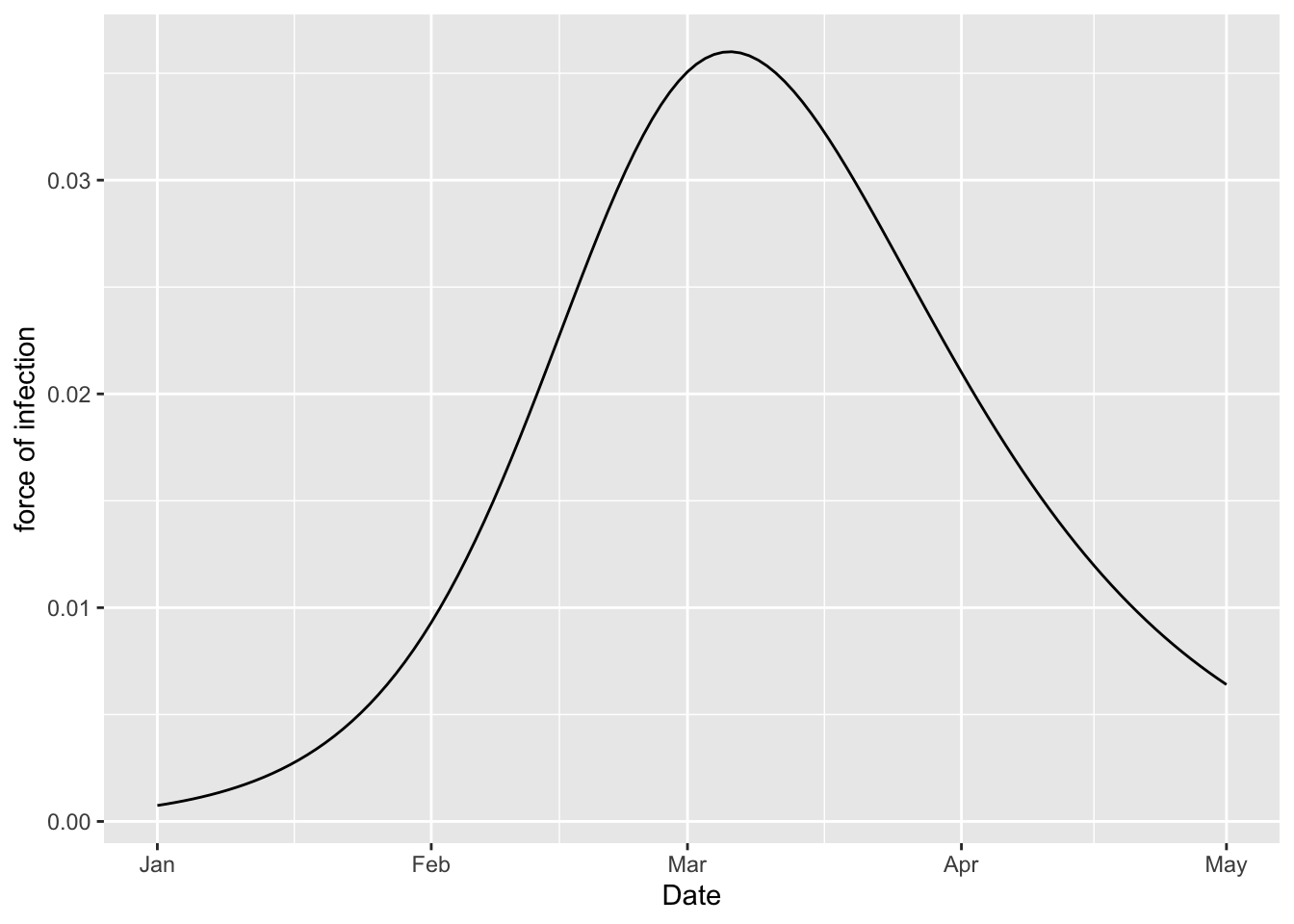One may use this functionality to compute basic epidemiological parameters such as $$R_0$$, $$r$$, and $$\bar{G}$$. We do this by modifying the model slightly, simulating, and summarizing the output. TODO: actually compute these parameters.

epi_pars(sir_model
, exposed_state_nm = "I"
, foi_nm = "S_to_I"
, N = 1
)

## 15.2 SI

si = (flexmodel(
params = c(beta = 0.15, gamma = 0.06, N = 100),
state = c(S = 99, I = 1),
start_date = "2000-01-01", end_date = "2000-06-01"
)
%>% add_rate("S", "I", ~ (I) * (beta) * (1/N))
%>% add_factr("ratio", ~ (gamma) * (1/beta))
%>% add_factr("S_hat", ~ (N) * (ratio))
%>% add_factr("I_hat", ~ (N) * (1 - ratio))
)
(si
%>% simulation_history
%>% select(-S_to_I, -ratio)
%>% pivot_longer(-Date, names_to = "State", values_to = "Population")
%>% separate(State, c("State", "Equilibrium"), "_")
%>% mutate(Equilibrium = ifelse(is.na(Equilibrium), 'no', 'yes'))
%>% ggplot
+ geom_line(aes(Date, Population, colour = State, linetype = Equilibrium))
)
## Warning: Expected 2 pieces. Missing pieces filled with NA in 306 rows [1, 2, 5, 6, 9,
## 10, 13, 14, 17, 18, 21, 22, 25, 26, 29, 30, 33, 34, 37, 38, ...].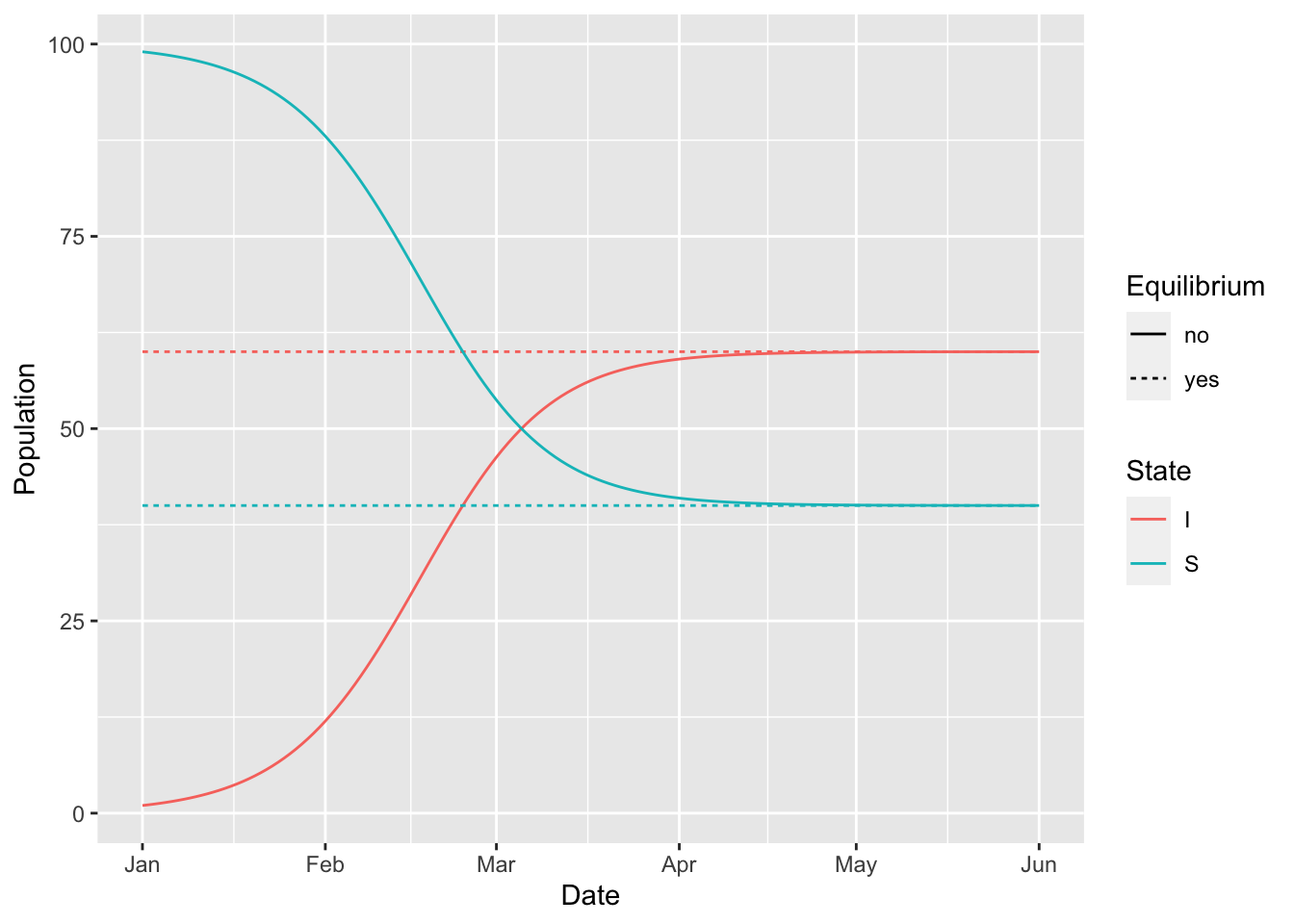## 15.3 SEIR

state = c(S = 20000, E = 0, I = 100, R = 0)
seir_model = (
flexmodel(
params = c(
alpha = 0.05,
gamma = 0.06,
beta = 0.15,
N = sum(state)
),
state = state,
start_date = "2000-01-01",
end_date = "2000-05-01",
do_hazard = TRUE,
)
%>% add_rate("S", "E", ~ (1/N) * (beta) * (I))
)
seir_model
##        from to n_fctrs n_prdcts n_vrbls state_dependent time_varying
## S_to_E    S  E       3        1       3            TRUE        FALSE
## E_to_I    E  I       1        1       1           FALSE        FALSE
## I_to_R    I  R       1        1       1           FALSE        FALSE
##        sum_dependent
## S_to_E         FALSE
## E_to_I         FALSE
## I_to_R         FALSE

## 15.4 Structure: Two-Strain SIR

strains = c("wild", "variant")
state = c(
S = 20000,
I_wild = 49, I_variant = 1,
R_wild = 0,   R_variant = 0
)
two_strain_model = (
flexmodel(
params = c(
gamma = 0.06,
beta_wild = 0.15,
beta_variant = 0.25,
N = sum(state)
),
state = state,
start_date = "2000-01-01",
end_date = "2000-05-01",
do_hazard = TRUE
)
%>% vec_rate(
"S",
"I" %_% strains,
vec("beta" %_% strains) * struc("1/N") * vec("I" %_% strains)
)
%>% rep_rate("I", "R", ~ (gamma))
)
regex = "^(S|I|R)(_*)(|wild|variant)$" (two_strain_model %>% simulation_history %>% select(Date, matches(regex)) %>% pivot_longer(!Date) %>% rename(state = value) %>% mutate(strain = sub(pattern = regex, replacement = "\\3", name)) %>% mutate(epi_cat = sub(pattern = regex, replacement = "\\1", name)) %>% mutate(strain = factor(strain, c("", "wild", "variant"))) %>% mutate(epi_cat = factor(epi_cat, c("S", "I", "R"))) %>% ggplot + geom_line(aes(x = Date, y = state, colour = epi_cat, linetype = strain)) )(two_strain_model %>% simulation_history %>% pivot_longer(starts_with("S_to_I")) %>% mutate(name = sub("S_to_I_", "", name)) %>% rename(force of infection = value) %>% rename(strain = name) %>% ggplot + geom_line(aes(x = Date, y = force of infection, colour = strain)) )## 15.5 Erlang SEIR David, Jonathan, and David describe the Erlang SEIR model in continuous time. Here is a discrete time version of it. n = 4 # number of I states m = 6 # number of E states erlang_seir = (flexmodel( # FIXME: only working for no demography (so mu = 0 for now) params = c(mu = 0, beta = 1.5, m = m, n = n, gamma = 1.2, sigma = 0.1), state = c( S = 1-1e-3, layered_zero_state("E" %_% 1:m), I_1 = 1e-3, layered_zero_state("I" %_% 2:n), R = 0, D = 0, . = 1), start_date = "2000-01-01", end_date = "2000-04-01", do_hazard = TRUE ) %>% add_state_param_sum("I", "^I_[0-9]+") # birth %>% add_rate(".", "S", ~ (mu)) # death %>% rep_rate( from = c("S", "E" %_% 1:m, "I" %_% 1:n, "R"), to = "D", formula = ~ (mu)) # infection %>% add_rate("S", "E_1", ~ (beta) * (I)) # become infectious %>% add_rate("E" %_% m, "I_1", ~ (m) * (sigma)) # sojourn through exposed compartments %>% rep_rate( "E" %_% 1:(m-1), "E" %_% 2:m, ~ (m) * (sigma) ) # sojourn through infectious compartments %>% rep_rate( "I" %_% 1:(n-1), "I" %_% 2:n, ~ (n) * (gamma) ) # recovery %>% add_rate("I" %_% n, "R", ~ (n) * (gamma)) # nothing flows out of . because it is a dummy # state used to generate flows that are not per-capita %>% add_outflow("[^.]") ) (erlang_seir %>% simulation_history %>% select(-., -D, -S_to_E_1) %>% pivot_longer(-Date, names_to = "State", values_to = "Count") %>% mutate(State = sub("_[0-9]+", "", State)) %>% group_by(Date, State) %>% summarise(Count = sum(Count)) %>% ungroup %>% mutate(State = factor(State, c("S", "E", "I", "R"))) %>% ggplot() + geom_line(aes(Date, Count, colour = State)) ) ## summarise() has grouped output by 'Date'. You can override using the ## .groups argument.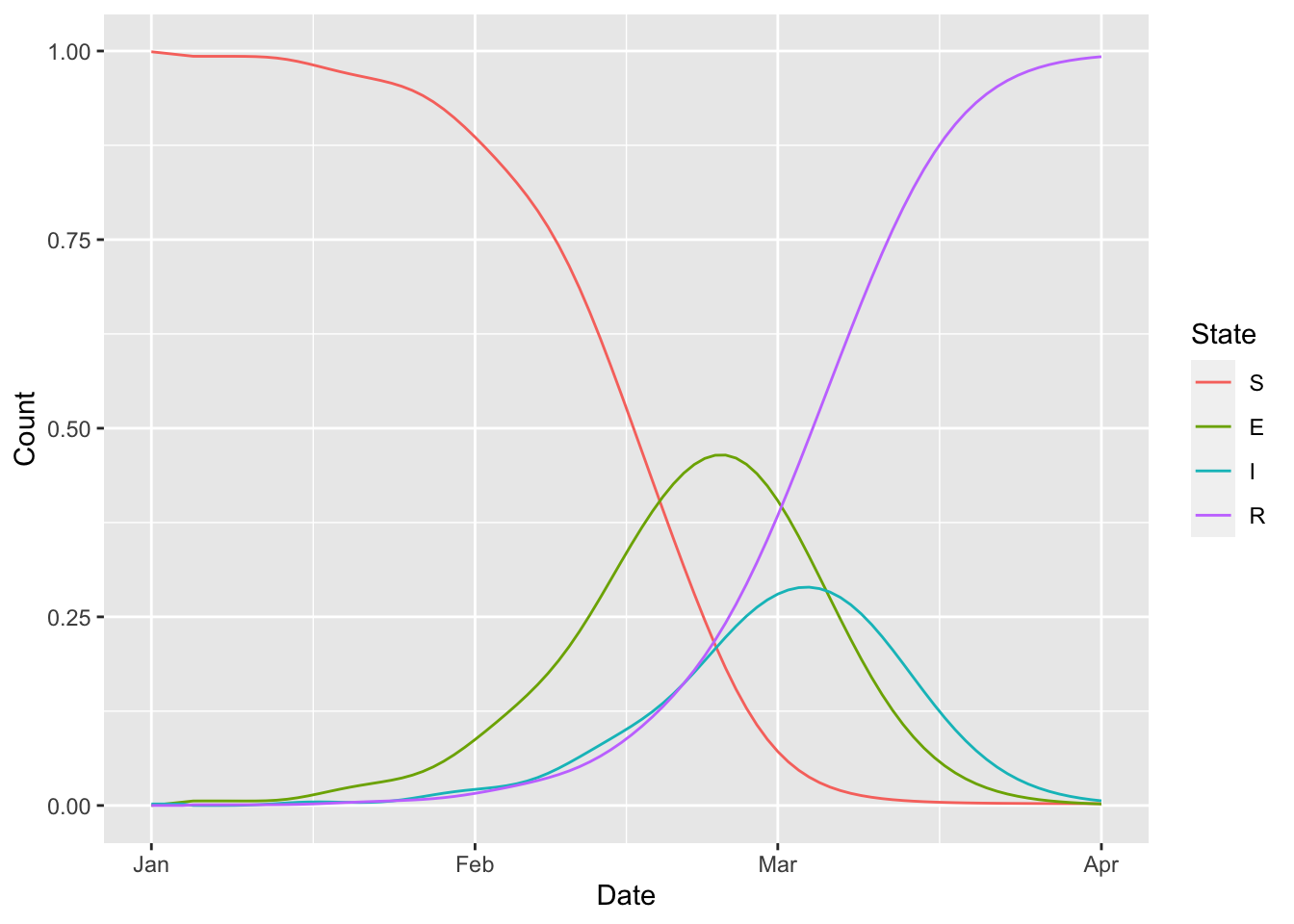# check to make sure that the population density # remains constant at one (erlang_seir %>% simulation_history %>% select(-Date, -., -D, -S_to_E_1, -I) %>% rowSums %>% sapply(all.equal, 1L) %>% sapply(isTRUE) %>% all ) ##  TRUE ## 15.6 SIRV state = c(S = 20000, I = 100, R = 0, V = 0) params = c( gamma = 0.06, beta = 0.15, v = 0, # initial vaccination rate N = sum(state) ) # roll out the vaccine by bumping the # vaccination rate twice params_timevar = data.frame( Date = c("2020-02-01", "2020-03-01"), Symbol = c("v", "v"), Value = c(0.01, 0.1), Type = c("abs", "abs")) sirv_model = ( flexmodel( params = params, state = state, params_timevar = params_timevar, start_date = "2020-01-01", end_date = "2020-05-01", do_hazard = TRUE ) %>% add_rate("S", "I", ~ (beta) * (1/N) * (I)) %>% add_rate("I", "R", ~ (gamma)) %>% add_rate("S", "V", ~ (v)) ) (sirv_model %>% simulation_history %>% select(Date, matches("^(S|I|R|V)$"))
%>% pivot_longer(!Date)
%>% rename(state = value, epi_cat = name)
%>% mutate(epi_cat = factor(epi_cat, topological_sort(sirv_model)))
%>% ggplot
+  geom_line(aes(x = Date, y = state, colour = epi_cat))
+  geom_vline(aes(xintercept = as.Date(Date)), data = params_timevar, colour = 'lightgrey')
)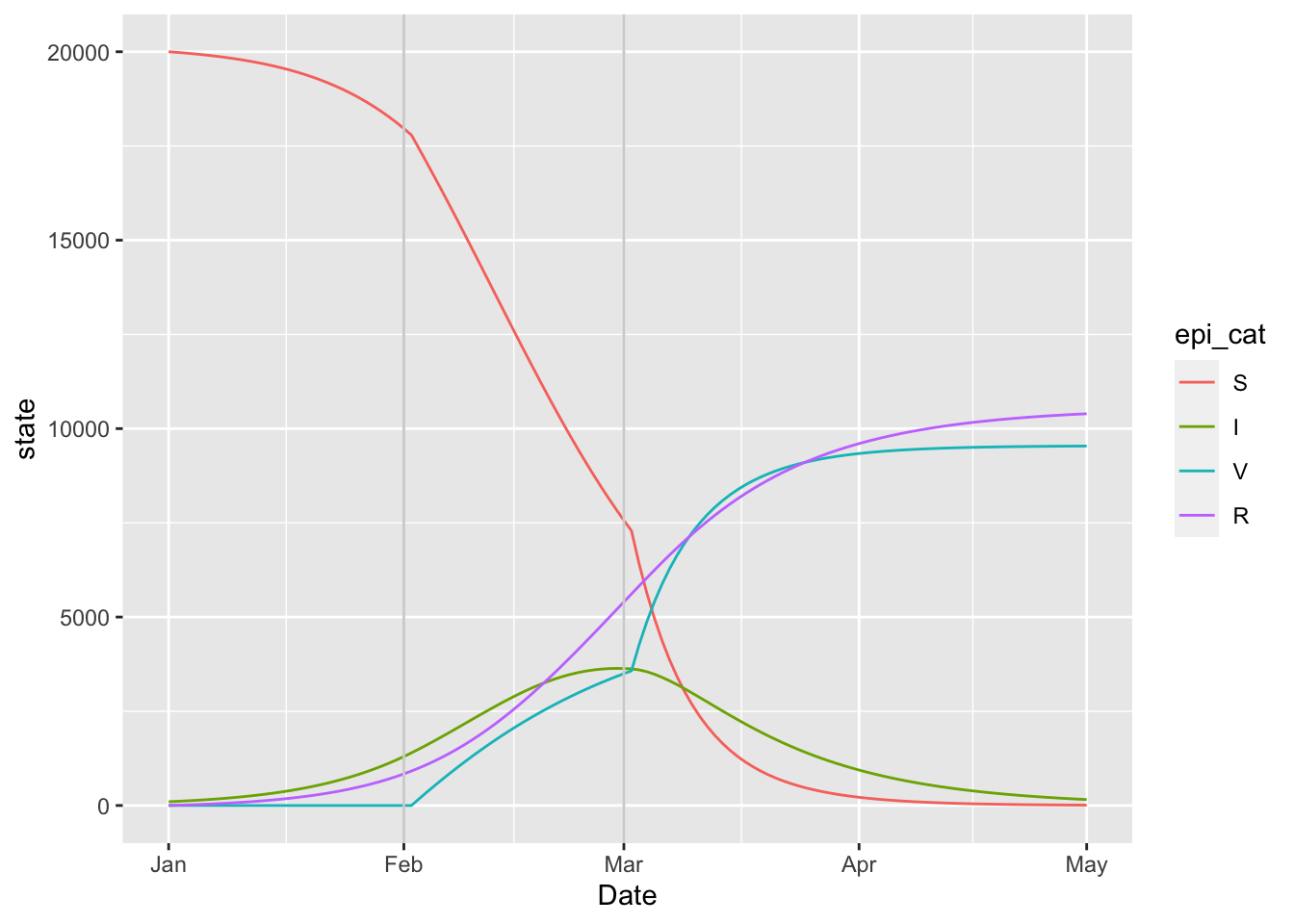## 15.7 Variolation model

The variolation model is … TODO: add description.

state = c(
S = 20000,
I_severe = 50, I_mild = 50,
R_mild = 0,   R_severe = 0
)
params = c(
nu = 0.0105, # birth rate
mu = 0.0105, # death rate
delta = 1/7, # waning immunity rate
gamma_mild = 1/(3.3 + 9),  # recovery rate of mild cases
gamma_severe = 1/(3.3 + 14), # recovery rate of severe cases
beta_mild = 0.15,  # transmission rate of infections leading to mild cases
beta_severe = 0.3, # transmission rate of infections leading to severe cases
m = 0.6 # probability of developing mild illness
)

# model structure
all_states = names(state)
severity = c("mild", "severe")
beta_vec = vec("beta" %_% severity)
I_vec = vec("I" %_% severity)
foi = kronecker(vec("m", "1-m"), sum(beta_vec * I_vec * struc("1/N")))

variolation_model <- (
flexmodel(
params = params,
state = state,
start_date = "2020-01-01",
end_date = "2020-04-01"
)

# births and deaths
# (FIXME: this only works if nu = mu)
%>% rep_rate(
all_states,
"S",
~ (nu)
)

# infection
%>% vec_rate(
"S",
"I" %_% severity,
foi
)

# waning immunity
%>% rep_rate(
"R" %_% severity,
"S",
~ (delta)
)

# recovery
%>% vec_rate(
"I" %_% severity,
"R" %_% severity,
vec("gamma" %_% severity)
)
)

(variolation_model
%>% simulation_history
%>% select(Date, matches(any_var(variolation_model$state))) %>% pivot_longer(!Date) %>% rename(state = value, epi_cat = name) %>% ggplot + geom_line(aes(x = Date, y = state, colour = epi_cat)) )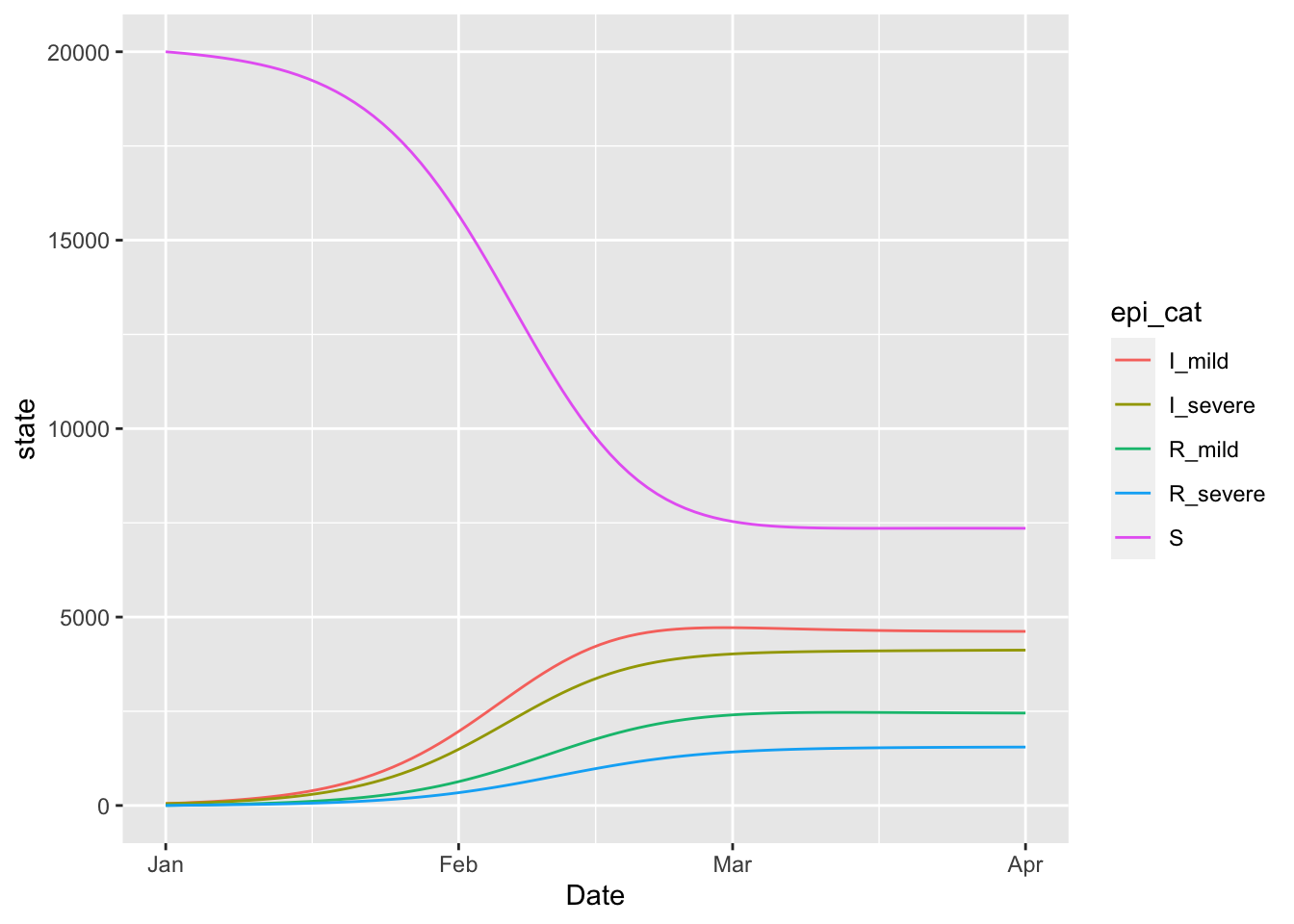## 15.8 SEIRD This is the Mac Theo Bio Model. state = c(S = 20000, E = 50, I = 50, R = 0, D = 0, Null = 0) params = c( # transmission rates of susceptibles from live and dead individuals beta_I = 5, beta_D = 2, # average times in various boxes T_E = 10, T_I = 14, T_D = 10, # probability of death given infection f = 0.5 ) seird_model = ( flexmodel( params = params, state = state, start_date = "2000-01-01", end_date = "2000-03-01", do_hazard = TRUE ) %>% add_state_param_sum("N", any_var(state)) %>% add_rate("S", "E", ~ (beta_I) * (1/N) * (I) + (beta_D) * (1/N) * (D)) %>% add_rate("E", "I", ~ (1/T_E)) %>% add_rate("I", "R", ~ (1/T_I) * (1 - f)) %>% add_rate("I", "D", ~ (1/T_I) * (f)) %>% add_rate("D", "Null", ~ (1/T_D)) ) (seird_model %>% simulation_history %>% select(Date, matches(any_var(state))) %>% pivot_longer(!Date) %>% rename(state = value, epi_cat = name) %>% mutate(epi_cat = factor(epi_cat, levels = topological_sort(seird_model))) %>% ggplot + geom_line(aes(x = Date, y = state, colour = epi_cat)) )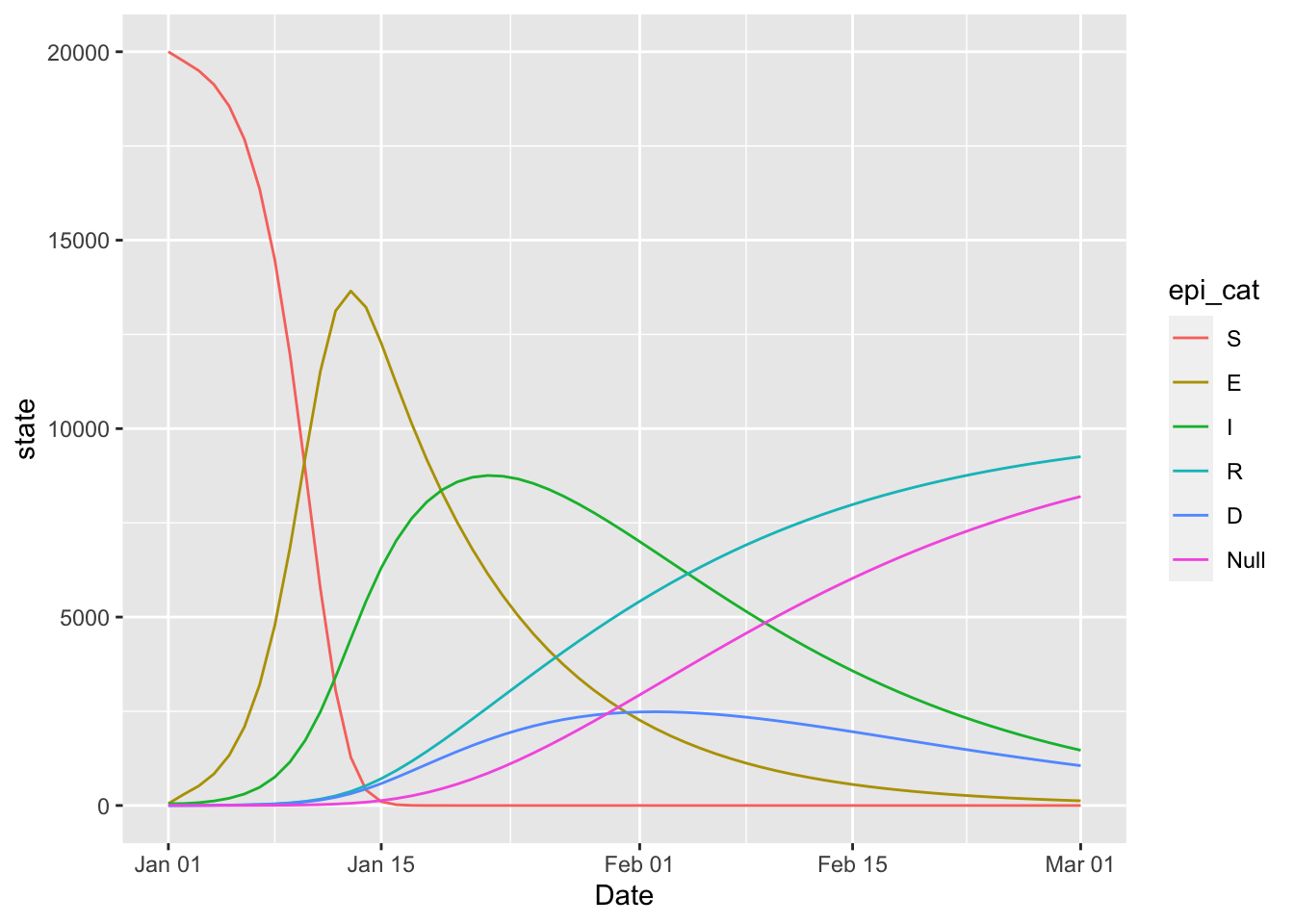## 15.9 Covid SEIR The BC covid modelling group uses this compartmental model for their inference and forecasting work. This model can be expressed in McMasterPandemic with the following. foi = ( vec(c("1", "f")) * struc("beta") * struc("1/N") * (struc("(I) + (E2)") + (struc("f") * struc("(Id) + (E2d)"))) ) params = c( N = 5100000, D = 5, k1 = 0.2, k2 = 1, q = 0.05, ud = 0.1, ur = 0.02, psir = 0.3, shape = 1.73, scale = 9.85, beta = 0.433, f = 1 ) state = c( S = 849999, E1 = 0.53, E2 = 0.13, I = 0.67, Q = 0, R = 0, Sd = 4249993, E1d = 2.67, E2d = 0.67, Id = 3.33, Qd = 0, Rd = 0 ) base_states = names(state)[1:6] dist_states = base_states %+% "d" strats = c("", "d") # distancing strategies ramp_period = seq(from = ymd(20210315), to = ymd(20210322), by = 1) time_ratio = rev((seq_along(ramp_period) - 1) / (length(ramp_period) - 1)) f2 = 0.22 params_timevar = data.frame( Date = ramp_period, Symbol = rep("f", length(ramp_period)), Value = f2 + time_ratio * (1 - f2), Type = rep("abs", length(ramp_period)) ) model = ( flexmodel( params = params, state = state, start_date = "2021-02-01", end_date = "2021-06-01", do_hazard = TRUE, params_timevar = params_timevar ) # flow between distancing strategies %>% rep_rate(base_states, dist_states, ~ (ud)) %>% rep_rate(dist_states, base_states, ~ (ur)) # force of infection %>% vec_rate( "S" %+% strats, "E1" %+% strats, foi ) # flow within distancing strategies %>% rep_rate( "E1" %+% strats, "E2" %+% strats, ~ (k1) ) %>% rep_rate( "E2" %+% strats, "I" %+% strats, ~ (k2) ) %>% rep_rate( "I" %+% strats, "Q" %+% strats, ~ (q) ) %>% rep_rate( expand_names(c("I", "Q"), strats, ""), expand_names(c("R", "R"), strats, ""), ~ (1/D) ) ) (model %>% simulation_history %>% select(Date, matches(any_var(model$state)))
%>% pivot_longer(!Date)
%>% ggplot()
+  facet_wrap(~ name, scales = 'free', ncol = 2)
+  geom_line(aes(Date, value))
)## 15.10 BC Covid Omicron

The BC group also developed a model with two strains for the Omicron wave of Covid-19.

DISCLAIMER: This is not meant to illustrate a realistic forecast, but rather to illustrate how the basic model structure can be expressed in McMasterPandemic

params = c(
sigma=1/3, # incubation period (3 days) (to fixed)
gamma=1/(5), #recovery rate (fixed)
nu =0.007, #vax rate: 0.7% per day (fixed)
mu=1/(82*365), # 1/life expectancy (fixed)
w1 = 1/(3*365),# waning rate from R to S (fixed)
w2 = 1/(3*365), # waning rate from Rv to V (fixed)
w3 = 1/(3*365),# waning rate Rw to W (fixed)
ve=1, # I think this should be 1. it is not really efficacy  ( fixed)
beta_r=0.72, #transmission rate (to estimate) (0.35)
beta_m=0.8*2.2, #transmission rate (to estimate)(*1.9)
epsilon_r = (1-0.8), # % this should be 1-ve
epsilon_m = (1-0.6), # % escape capacity #(fixed)
b= 0.006, # booster rate  (fixed)
beff = 0.7, # booster efficacy
wf=0.2, # protection for newly recoverd #0.2
N=5e6,
E0=5,
S0=1-1e-5,
c=1
)

# dimensions of model structure
vax_states = c("unvax", "onedose", "boost")
variant_states = c("delta", "omicron")
epi_states = c("S", "E" %_% variant_states, "I" %_% variant_states, "R")

# vectors representing variant model structure
I_delta   = vec("I" %_% "delta"   %_% vax_states)
I_omicron = vec("I" %_% "omicron" %_% vax_states)
E_delta   = vec("E" %_% "delta"   %_% vax_states)
E_omicron = vec("E" %_% "omicron" %_% vax_states)

# initial state vector
state = layered_zero_state(epi_states, vax_states)
all_states = names(state)
state[] = unname(make_init(params))
print(state)
##           S_unvax     E_delta_unvax   E_omicron_unvax     I_delta_unvax
##      9.106607e+05      6.480000e+02      1.748571e+01      1.110857e+03
##   I_omicron_unvax           R_unvax         S_onedose   E_delta_onedose
##      2.997551e+01      1.014000e+05      3.502789e+06      5.924571e+02
## E_omicron_onedose   I_delta_onedose I_omicron_onedose         R_onedose
##      1.974857e+01      1.015641e+03      3.385469e+01      3.893760e+05
##           S_boost     E_delta_boost   E_omicron_boost     I_delta_boost
##      1.460132e+05      2.468571e+01      8.228571e-01      4.231837e+01
##   I_omicron_boost           R_boost
##      1.410612e+00      1.622400e+04
two_strain_bc = (flexmodel(
params = params,
state = state,
start_date = "2022-01-01",
end_date = "2022-05-01",
do_hazard = TRUE
)

# sum over every vax status to get
# total numbers in I boxes for each
# variant

# R to S boxes for every vax status
#   -- waning
%>% vec_rate(
from = "R" %_% vax_states,
to   = "S" %_% vax_states,
vec('w1', 'w2', 'w3')
)

# S_delta to E_delta for every vax status
#   -- delta force of infection
%>% vec_rate(
from = "S"             %_% vax_states,
to   = "E" %_% "delta" %_% vax_states,
struc("(c) * (beta_r) * (1/N) * (I_delta)") * vec("1", "epsilon_r", "epsilon_r")
)

# S_omicron to E_omicron for every vax status
#   -- omicron force of infection
%>% vec_rate(
from = "S"               %_% vax_states,
to   = "E" %_% "omicron" %_% vax_states,
struc("(c) * (beta_m) * (1/N) * (I_omicron)") * vec("1", "epsilon_m", "epsilon_m")
)

# R to E_delta for every vax status
#   -- delta force of infection of recovered individuals
%>% rep_rate(
from = "R"             %_% vax_states,
to   = "E" %_% "delta" %_% vax_states,
~ (wf) * (epsilon_r) * (c) * (beta_r) * (1/N) * (I_delta)
)

# R to E_omicron for every vax status
#   -- omicron force of infection of recovered individuals
%>% rep_rate(
from = "R"               %_% vax_states,
to   = "E" %_% "omicron" %_% vax_states,
~ (wf) * (epsilon_m) * (c) * (beta_m) * (1/N) * (I_omicron)
)

# E to I for all variant-vax combinations
%>% rep_rate(
from = "E" %_% expand_names(variant_states, vax_states),
to   = "I" %_% expand_names(variant_states, vax_states),
~ (sigma)
)

# recovery for all variant-vax combinations
%>% rep_rate(
from = "I" %_% expand_names(variant_states, vax_states),
to   = "R" %_% rep(vax_states, each = length(variant_states)),
~ (gamma)
)

# demographics
%>% rep_rate(
from = all_states,
to   = "S_unvax",
~ (mu)
)

# vaccination
%>% add_rate(from = "S_unvax",   to = "S_onedose", ~ (nu) * (ve))
%>% add_rate(from = "S_onedose", to = "S_boost",   ~ (b)  * (ve))
)

category_pattern = "^(S|E|I|R)(_omicron|_delta)?_(unvax|onedose|boost)\$"
(two_strain_bc
%>% simulation_history
%>% select(Date, matches(category_pattern))
%>% pivot_longer(-Date, names_to = "Compartment", values_to = "State")
%>% mutate(Epi Status = sub(category_pattern, '\\1\\2', Compartment, perl = TRUE))
%>% mutate(Vaccination Status = sub(category_pattern, '\\3', Compartment, perl = TRUE))
%>% arrange(Epi Status, Vaccination Status)
%>% mutate(Compartment = factor(Compartment, all_states))
%>% ggplot()
+  facet_wrap(
~ Compartment, ncol = 3, scales = 'free', dir = 'v')
+  geom_line(aes(Date, State))
)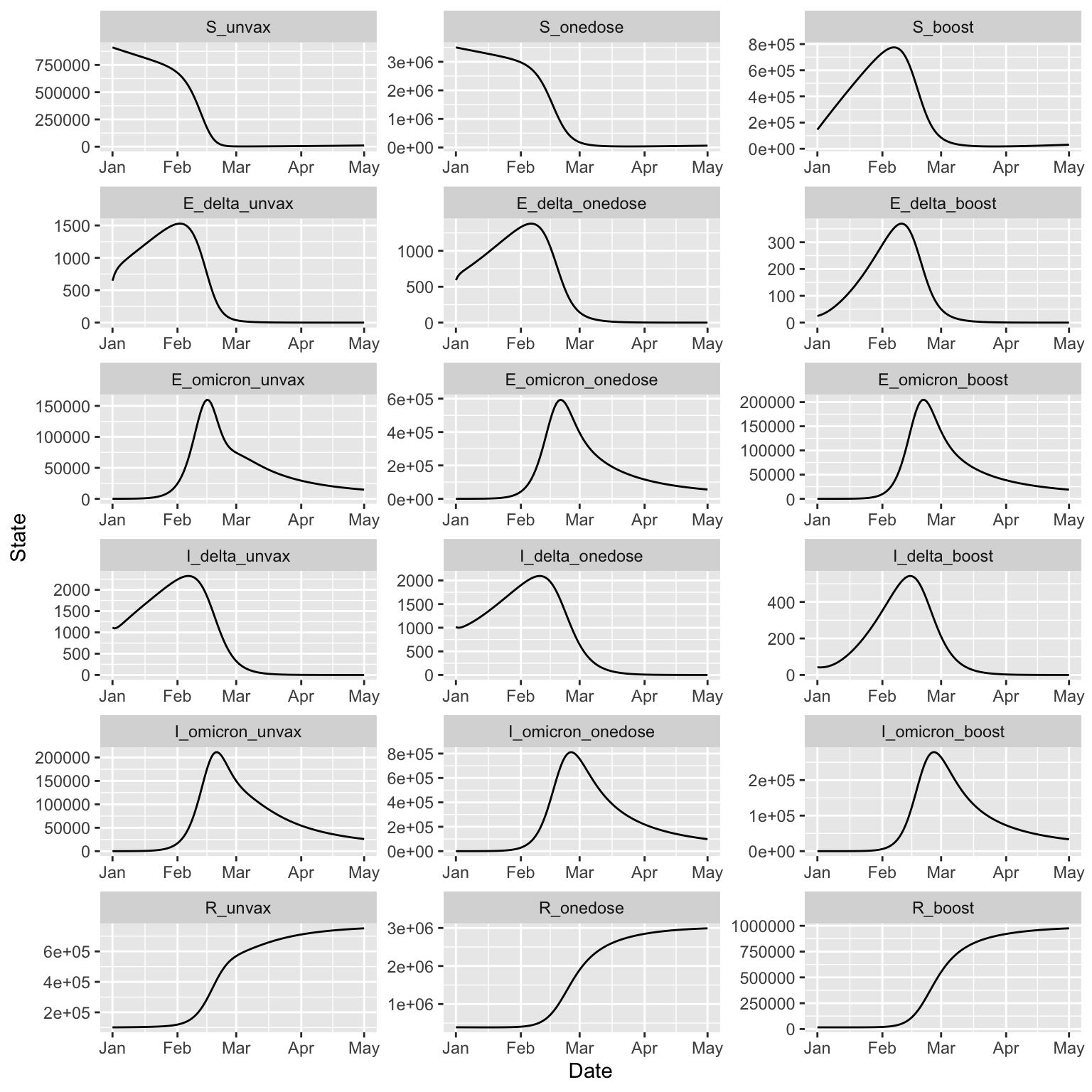## 15.11 Classic McMasterPandemic

params = read_params("ICU1.csv")
model = (flexmodel(
params = params,
state = make_state(params = params),
start_date = "2020-03-10",
end_date = "2020-12-10",
do_make_state = FALSE,
do_hazard = TRUE
)
%>% add_rate("E", "Ia", ~ (alpha) * (sigma))
%>% add_rate("E", "Ip", ~ (1 - alpha) * (sigma))
%>% add_rate("Ip", "Im", ~ (mu) * (gamma_p))
%>% add_rate("Ip", "Is", ~ (1 - mu) * (gamma_p))
(1 - nonhosp_mort) * (phi1) * (gamma_s))
(1 - nonhosp_mort) * (1 - phi1) * (1 - phi2) * (gamma_s))
(1 - nonhosp_mort) * (1 - phi1) * (phi2) * (gamma_s))
%>% add_rate("Is", "D", ~ (nonhosp_mort) * (gamma_s))
%>% add_rate("Is", "X", ~ (1 - nonhosp_mort) * (phi1) * (gamma_s))
(Ia) * (beta0) * (1 / N) * (Ca) +
(Ip) * (beta0) * (1 / N) * (Cp) +
(Im) * (beta0) * (1 / N) * (Cm) * (1 - iso_m) +
(Is) * (beta0) * (1 / N) * (Cs) * (1 - iso_s))
)
(model
%>% simulation_history
%>% pivot_longer(!Date)
%>% mutate(name = factor(name, levels = topological_sort(model)))
%>% ggplot()
+  facet_wrap(~ name, scales = 'free', ncol = 3)
+  geom_line(aes(Date, value))
)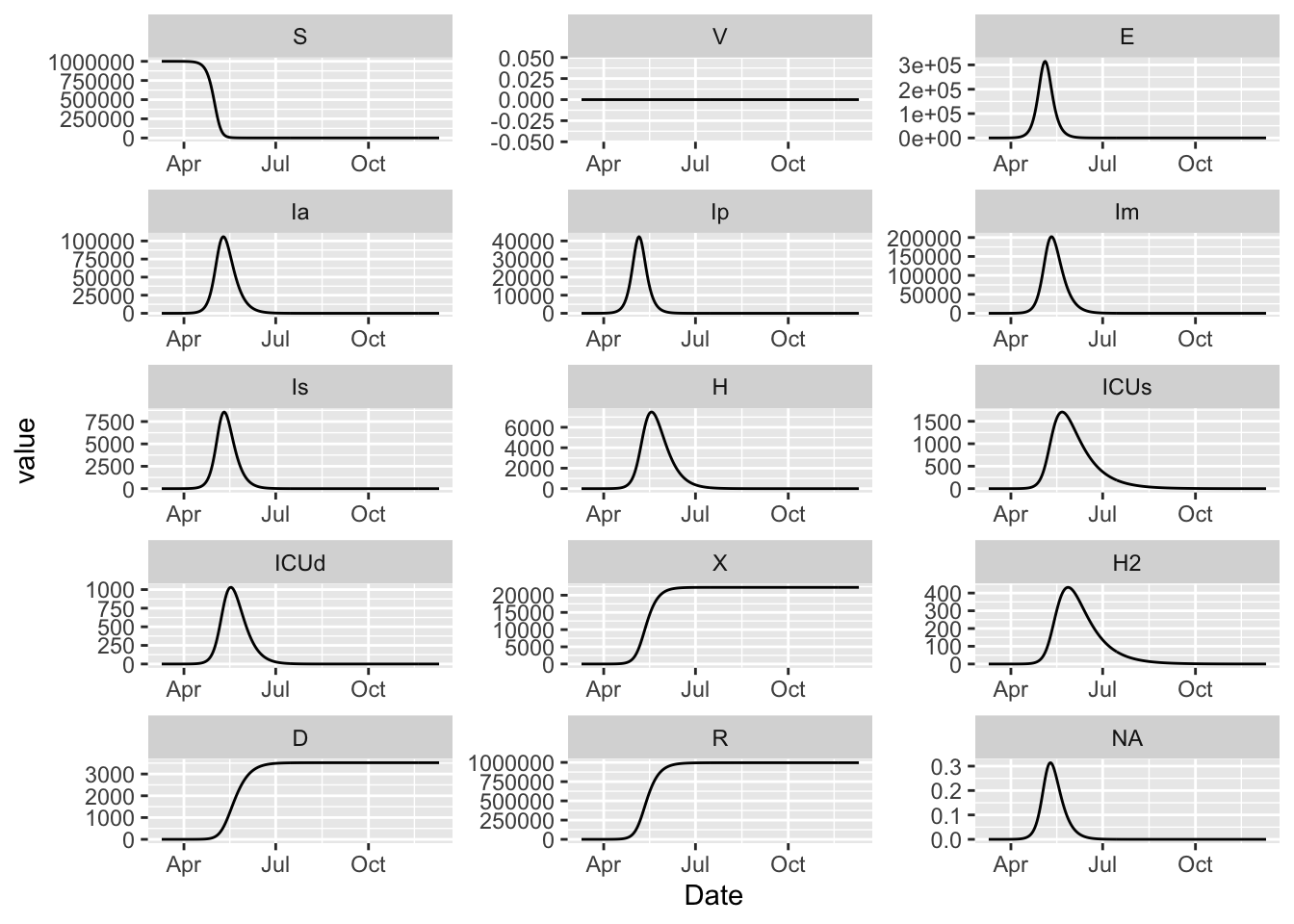## 15.12 Granich HIV Model

The HIV model from Granich et al (2008).

start_date = ymd(20220501)
end_date = start_date + days(30)

params = c(
# phenomenological heterogeneity parameters and constants
alpha = 0.1,
lambda0 = 0.002,
n = 1,  # exponential decrease when n = 1
e = exp(1),
minus_1 = -1,

# background birth and mortality rates
beta = 0.02,
mu = 0.01,
one = 1,

# treatment efficacy (smaller is less infectious)
epsilon = 0.2,

# disease progression rate
rho = 0.15,

# treatment rate
tau = 0.8, # value from paper

# treatment stopping rate
phi = 0.015, # value from paper

# disease progression rate for treated individuals
sigma = 0.1
)

number_of_stages = 4
state = c(
S = 999,
layered_zero_state("I", 1:number_of_stages),
layered_zero_state("A", 1:number_of_stages),
D_disease = 0,
D_background = 0
#birth_pool = 1000
)
state["I_1"] = 1

alive_state_nms = grep("^(S|I|A)", names(state), value = TRUE)
Ivec = vec("I" %_% 1:number_of_stages)
Avec = vec("A" %_% 1:number_of_stages)
epsilon = struc("epsilon")
I = as.character(sum(Ivec) + sum(Avec))
J = as.character(sum(Ivec) + sum(epsilon * Avec))

granich = (flexmodel(
params = params,
state = state,
start_date = start_date,
end_date = end_date,
do_hazard = TRUE
)
%>% add_factr("minus_alpha", ~ (alpha) * (minus_1))
%>% add_factr("P", ~ (I) * (1/N))

%>% rep_rate(
from = alive_state_nms,
to   = "S",
~ (beta)
)

%>% rep_rate(
from = alive_state_nms,
to = "D_background",
~ (mu)
)

# transmission
from = "S",
to = "I_1",
~ (lambda) * (S) * (J) * (1/N)
)

# treatment dynamics
%>% rep_rate(
from = "I" %_% (1:number_of_stages),
to   = "A" %_% (1:number_of_stages),
~ (tau)
)
%>% rep_rate(
from = "A" %_% (1:number_of_stages),
to   = "I" %_% (1:number_of_stages),
~ (phi)
)

# disease progression
%>% rep_rate(
from = "I" %_% (1:(number_of_stages - 1)),
to   = "I" %_% (2:number_of_stages),
~ (rho)
)
%>% rep_rate(
from = "A" %_% (1:(number_of_stages - 1)),
to   = "A" %_% (2:number_of_stages),
~ (sigma)
)

# disease death
%>% add_rate("I" %_% number_of_stages, "D_disease", ~ (rho))
%>% add_rate("A" %_% number_of_stages, "D_disease", ~ (sigma))

#%>% add_outflow(from = "^[A-Z]") # don't flow out of the 'birth pool'
from = '.+',
to = '^(I|A|D)_'
)

)